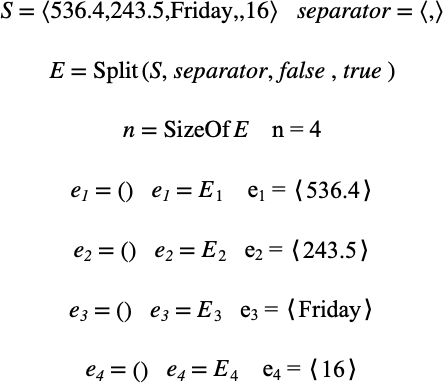# $$\text{Split}$$¶

You can use the $$\text{Split}$$ function to split a tuple into multiple smaller tuples. The $$\text{Split}$$ function is primarily intended to be used for text strings although it can be used for tuples containing arbitrary data.

You can use the \split backslash command to insert this function.

The following variants of this function are available:

• $$\text{tuple } \text{Split} \left ( \text{<tuple>}, \text{<split at>} \right )$$

• $$\text{tuple } \text{Split} \left ( \text{<tuple>}, \text{<split at>}, \text{<keep separators>} \right )$$

• $$\text{tuple } \text{Split} \left ( \text{<tuple>}, \text{<split at>}, \text{<keep separators>}, \text{<remove empty>} \right )$$

The $$\text{Split}$$ function returns a tuple containing tuples holding each subsection of the original $$\text{<tuple>}$$ entry.

The $$\text{<tuple>}$$ parameter is the tuple to be separated into parts. The $$\text{<split at>}$$ parameter is a tuple holding the entries to use to identify the separator.

The $$\text{<keep separators>}$$ parameter is an optional boolean value indicating if the separator entries should be included in the returned tuples. A value of true will cause the separators to be included. A value of false will cause the separators to be removed from the returned tuples. If not included in the parameter list, separators will be removed.

The $$\text{<remove empty>}$$ parameter is an optional boolean value indicating if zero length tuples should be excluded from the returned value. A value of true indicates that empty tuples should be removed. A value of false indicates that empty tuples should be included. If not included in the parameter list, empty tuples will be included.

The Figure 205 shows how you can use the $$\text{Split}$$ function.Figure 205 Example Use Of The Split Function